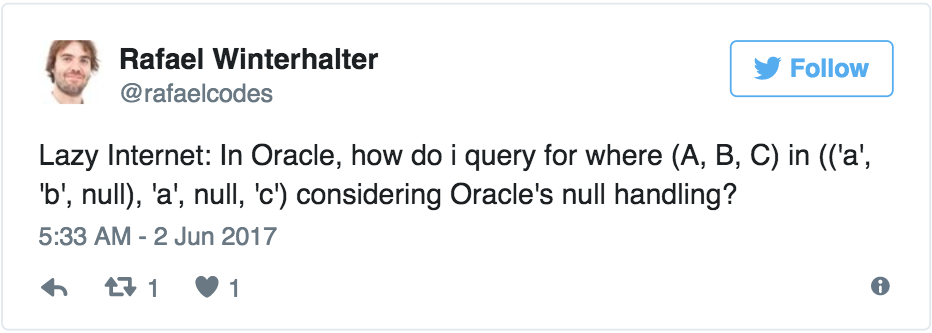{{announcement.body}}
{{announcement.title}}

# How to Use SQL INTERSECT to Work Around SQL's NULL Logic

DZone 's Guide to

# How to Use SQL INTERSECT to Work Around SQL's NULL Logic

### Stumped at how to get around Oracle's NULL logic when searching for items in a query using 'IN'? In this post, we see if we can't figure out how to make this work.

· Database Zone ·
Free Resource

Comment (0)

Save
{{ articles.views | formatCount}} Views

Another SQL post this week? I got nerd-sniped:Oooooh, challenge accepted!

So, let’s assume we have a table `T` with columns `(A, B, C)` like this:

``````WITH t(a, b, c) AS (
SELECT 'a', 'b', null FROM dual UNION ALL
SELECT 'a', null, 'c' FROM dual UNION ALL
SELECT 'a', 'b', 'c'  FROM dual
)
SELECT * FROM t``````

As expected, this yields:

``````A       B       C
-----------------
a       b
a               c
a       b       c``````

Truly exciting.

Now, we want to find all those rows that “match” either `('a', 'b', NULL)` or `('a', NULL, 'b')`. Clearly, this should produce the first two rows, right?

``````A       B       C
-----------------
a       b
a               c``````

Yes. Now, the canonical solution would be to tediously write out the entire predicate as such:

``````WITH t(a, b, c) AS (...)
SELECT * FROM t
WHERE (a = 'a' AND b = 'b' AND c IS NULL)
OR (a = 'a' AND b IS NULL AND c = 'c')``````

That’s really boring. Sure, we could have factored out the first, common predicate:

``````WITH t(a, b, c) AS (...)
SELECT * FROM t
WHERE a = 'a' AND (
(b = 'b' AND c IS NULL)
OR (b IS NULL AND c = 'c')
)``````

That’s certainly better from a performance perspective, but Rafael had a nifty idea. Let’s use row value expressions (tuples) in our predicates:

``````WITH t(a, b, c) AS (...)
SELECT * FROM t
WHERE (a, b, c) IN (('a', 'b', NULL), ('a', NULL, 'c'))``````

Unfortunately, this doesn’t yield any results because nothing is equal to `NULL` in SQL (not even `NULL` itself). The above query is the same as this one:

``````WITH t(a, b, c) AS (...)
SELECT * FROM t
WHERE (a = 'a' AND b = 'b' AND c = NULL /* oops */)
OR (a = 'a' AND b = NULL /* oops */ AND c = 'c')``````

D’oh.

## Solutions

We've got a couple...

### The Lame One

The canonical solution then would be a really lame (but perfectly valid) one. Encode `NULL` to be some “impossible” string value. Rafael suggested `yolo`. Fair enough.

This works:

``````WITH t(a, b, c) AS (...)
SELECT * FROM t
WHERE (a, NVL(b, 'yolo'), NVL(c, 'yolo'))
IN (('a', 'b', 'yolo'), ('a', 'yolo', 'c'))``````

All we have to do now is to always remember the term `yolo` which means “`NULL`, but not `NULL` thank you SQL.”

### The Hipster One

But wait! SQL is painstakingly inconsistent when it comes to `NULL`. See, `NULL` really means `UNKNOWN` in three-valued logic, and this means, we never know if SQL abides to its own rules.

Come in `INTERSECT`. Like `UNION` or `EXCEPT` (`MINUS`) in Oracle, as well as `SELECT DISTINCT`, these set operations handle two `NULL` values as `NOT DISTINCT`. Yes, they’re not equal but also not distinct. Whatever. Just remember: That’s how it is

So, we can write this hipster solution to Rafael’s problem:

``````WITH t(a, b, c) AS (...)
SELECT *
FROM t
WHERE EXISTS (
SELECT a, b, c FROM dual
INTERSECT (
SELECT 'a', 'b', null FROM dual
UNION ALL
SELECT 'a', null, 'c' FROM dual
)
)``````

We create an intersection of the tuple `(a, b, c)`, the left side of Rafael’s `IN` predicate, and the desired values on the right side of the `IN` predicate, and we’re done.

Clearly less tedious than writing the original predicates, right? (We won’t look into performance this time.)

Topics:
database, null, oracle, sql, tutorial

Comment (0)

Save
{{ articles.views | formatCount}} Views

Published at DZone with permission of Lukas Eder , DZone MVB. See the original article here.

Opinions expressed by DZone contributors are their own.